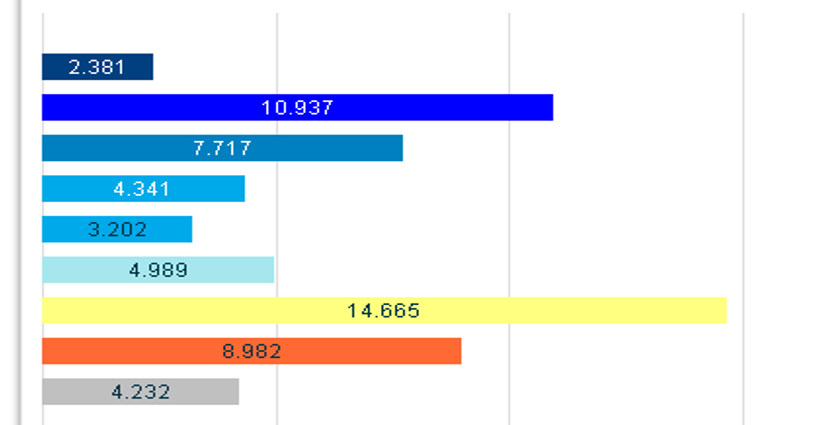# New to QlikView

Discussion board where members can get started with QlikView.

Announcements
Qlik Analytics Tour 2020 Online. Begins August 10th. Register Today
cancel
Showing results for
Did you mean:
HighlightedCreator

## Help with aggr dimension

Hi! community.

759/5000

Tags (4)
1 Solution

Accepted Solutions
HighlightedMVP

## Re: Help with aggr dimension

For single value the total qualifier should work...

=Count (TOTAL DISTINCT Aggr(If((Sum({<CHARGE_TYPE_DESC = {'Credit'}>} CHARGE_NET_AMOUNT) + Sum({<CHARGE_TYPE_DESC = {'Discount'}>} CHARGE_NET_AMOUNT))

/
Sum({<CHARGE_TYPE_DESC = {'Debit'}>} CHARGE_NET_AMOUNT) >= 0.8 and
Sum(CHARGE_NET_AMOUNT) >= 1, INVOICE_KEY), INVOICE_KEY))

But for multiple values, which field or fields would you want the count on?

5 Replies
HighlightedMVP

## Re: Help with aggr dimension

What exactly are you hoping to see in your dimension? A single value or bunch of values?

HighlightedCreator

## Re: Help with aggr dimension

In this case a single value,

but I would appreciate if you guide me for when it comes to a bunch of values.

Thanks

HighlightedMVP

## Re: Help with aggr dimension

For single value the total qualifier should work...

=Count (TOTAL DISTINCT Aggr(If((Sum({<CHARGE_TYPE_DESC = {'Credit'}>} CHARGE_NET_AMOUNT) + Sum({<CHARGE_TYPE_DESC = {'Discount'}>} CHARGE_NET_AMOUNT))

/
Sum({<CHARGE_TYPE_DESC = {'Debit'}>} CHARGE_NET_AMOUNT) >= 0.8 and
Sum(CHARGE_NET_AMOUNT) >= 1, INVOICE_KEY), INVOICE_KEY))

But for multiple values, which field or fields would you want the count on?

HighlightedCreator

## Re: Help with aggr dimension

Thanks Sunny

The operation

sum ({<CHARGE_TYPE_DESC = {'Credit'}>} CHARGE_NET_AMOUNT)

+

sum ({<CHARGE_TYPE_DESC = {'Discount'}>} CHARGE_NET_AMOUNT) )

/

sum ({<CHARGE_TYPE_DESC = {'Debit'}>} CHARGE_NET_AMOUNT)

it can be too between:

>0.01  - < 0.20

> 0.20 and <0.40

> 0.40 and <0.80

> 0.80 (its okey, already )

= 100

Sum(CHARGE_NET_AMOUNT) >= 1, INVOICE_KEY don't change it stays the same

HighlightedCreator

## Re: Help with aggr dimension

Hi Sunny,

I have this other example:

I got a bar char that contains 7 expressions, each one for a brunch of values. The Bar chart dont have a dimenssion and really,,, i don't like it.. I would like to do better

The detail for each bar it is:

1 Expression: 70 - 75

=Count(DISTINCT Aggr(If(Sum(

CHARGE_NET_AMOUNT) <= 74.9 AND Sum(CHARGE_NET_AMOUNT) >= 70 , INVOICE_KEY), INVOICE_KEY))

2. Expression: 60-69

=Count(DISTINCT Aggr(If(Sum(CHARGE_NET_AMOUNT) <= 69.9 AND Sum(CHARGE_NET_AMOUNT) >= 60 , INVOICE_KEY), INVOICE_KEY))

3. Expression: 50-59

=Count(DISTINCT Aggr(If(Sum( CHARGE_NET_AMOUNT) <= 59.9 AND Sum(CHARGE_NET_AMOUNT) >= 50 , INVOICE_KEY), INVOICE_KEY))

..And so on until reach the last expression.

The Bar chart i've created looks like:I wanted to create an adequate dimension to simplify brunch of values expressions and can counting the numbers of Invoice..

from now thank you very much if you can help mestalwar1